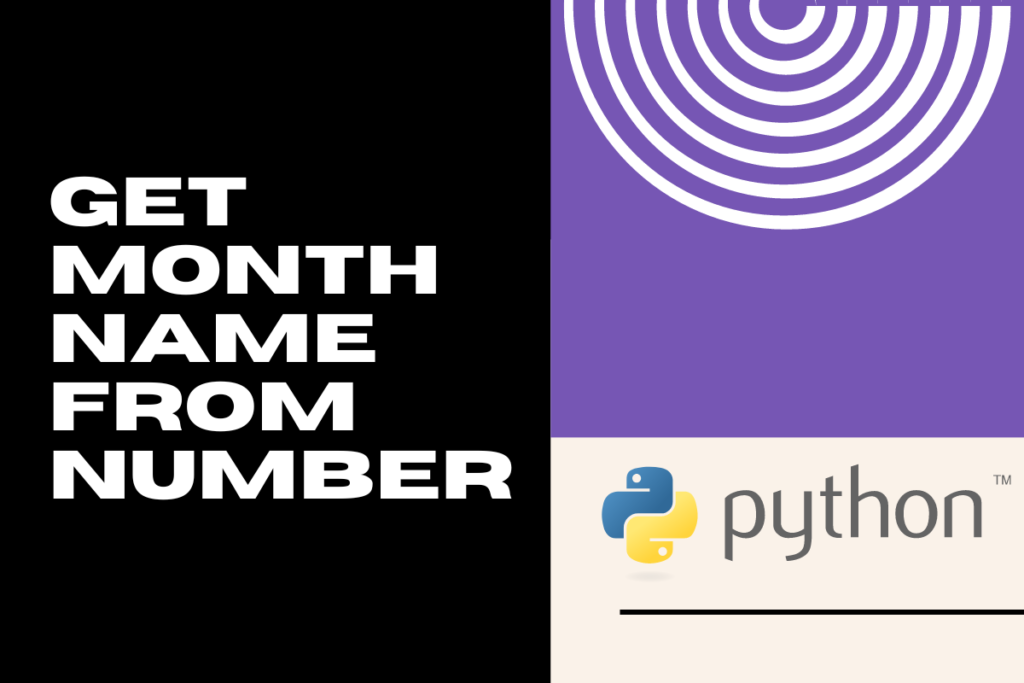# How to Get Month Name from Number in Python?Sometimes numbers just are not enough. More and more numbers make it a tad bit difficult to differentiate and interpret data. That’s exactly where textual information comes in! A bit of textual data goes a long way in explaining what those numbers stand for. At times, one might also see oneself in a situation where the numbers ought to be converted into textual data.

This article sets out to explore how the conversion of numbers into the names of months could be done in Python. It elaborates further on the different techniques & functions there are, from which the one that bests suits could be used. Listed below are the different methods to return month names from a number in Python.

• Using calendar module
• Using datetime module
• Using pandas module

## Method I – Using the calendar module

One shall get started by importing the calendar module in Python using the below code.

```import calendar
```

Thereafter, one shall assign a variable which is mapped with a number for which the month name is to be returned.

```m_num = 5
```

If the requirement is to return the full name of the month, one shall use the calendar.month_name[ ] function as shown below.

```print ('Month name for',m_num,'is',calendar.month_name[m_num])
```

Following is the result when the above code is run.

What if one wants to keep the name short? What then? The calendar module also provides a way around this too! When the calendar.month_abbr[ ] is used, only the short format of the month name will be returned. The following code demonstrates better.

```m_num = 9
print ('Output:\nMonth name for',m_num,'is',calendar.month_abbr[m_num])
```

## Method II – Using the datetime module

Here’s another technique that can be used to return the month name from a given number & it involves the usage of a function from the datetime module – the strftime( ) function. Similar to that of the calendar module, both the short and long names of the month can be obtained by using the strftime( ) function too.

So, let us get started by importing the datetime module as shown below.

```from datetime import datetime
```

The datetime module has been imported in such a way as to enable one to go further. One might as well go the extra mile with the datetime module by distilling the month name directly from the date, rather than feeding in numbers for the month name conversion. Let us take the following example where the month shall be extracted from the current date using the now( ) function.

```c_date = datetime.now()
print('Current Date: ', c_date)
```

Once done one can use the strftime( ) function to return both the short & full names of the months. Yep! When in need of the month name in full, use the ‘%B’, whereas ‘%b’ is to be used when the short name of the month is needed. Both have been put into use for demonstration in the below code.

```print('Current Month Number: ', c_date.month)
print('Month Short Name: ', c_date.strftime('%B'),
'\nMonth Full Name: ', c_date.strftime('%b'))
```

Following is the result when the aforementioned lines of code are run.

## Method III – Using the pandas module

If one has a series of dates for which the month name of each date is to be returned then one might very well use the dt.month_name( ) function from the pandas module to make it happen! Following is a series of dates.

```import pandas as pd
dates = pd.Series(pd.date_range(start='2023-01-01',freq='W', periods=10))
print(dates)
```

Now to return the month names, the following code is used.

```print('Month Names:\n',dates.dt.month_name( ))
```